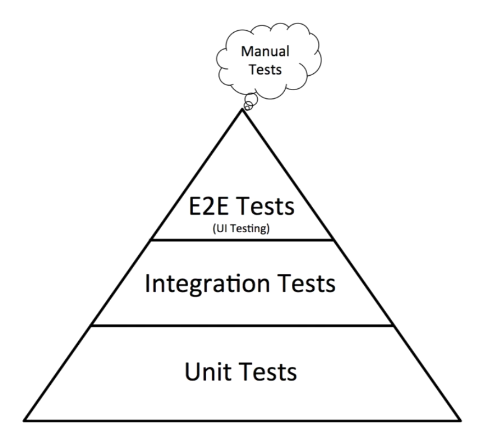# Testing Ruby with RSpec: The Complete Guide

#ruby

[TOC]

## Section 1: Introduction

### Types of Tests

Three layers of tests:

• Unit tests: focus on individual units (a class, module, object or method)
• Integration tests:
• E2E tests: focus on a feature and its interaction with the entire system.
• specs hard to write, hard to troubleshoot and run slow.

We should have more unit tests, then integration tests, and then end-to-end tests.I also like what Kent Dods says in https://testingjavascript.com:### Install RSpec and Start a Project

Install globally:

``````gem install rspec
``````

Start a new project

``````mkdir rspec-course
cd  rspec-course
rspec --init
``````

The "init" will create `.rspec` and `spec/spec_helper.rb`

### Test-Driven Development TDD

It's a top-down approach, where you first think about how you wanna use a piece of software, and then write the software.

Red -> Green -> Refactor

```graph LR
Red --> Green --> Refactor --> Red```

What are the benefits of TDD?

It forces you to become a better developer. Simply practicing this thing is one of the best ways that I have become a better developer and matured as a programmer, especially in my object oriented thinking.
You don't have to read additional blog posts. This is something that you can do every day. Whenever you write code, just write your tests first.

### The `describe` method - example group

The `describe` method creates an example group.

``````RSpec.describe 'Card' do

end
``````
• RSpec is a module
• on that module we have the `describe` method
• we give two arguments to the `describe` method:
• the `'Card'` string
• a `do-end` block
• inside the `do-end` block is where we will write all of our tests for the `Card`.

A test is also known as an "example". And "example group" is a set of related tests.

### The `it` method - a single example

The `describe` creates an example group, the `it` method creates a single example.

``````RSpec.describe 'Card' do
it 'has a type' do

end
end
``````

The idea is to describe how the software should behave, instead of saying how it should be implemented.

Note: the `specify` method has the exact same meaning as `it`.

### The `expect` method

Doing assertions with `expect`.

``````RSpec.describe 'Card' do
it 'has a type' do
end
end
``````

### Assignment 1

Create an example group with a string argument of "math calculations".

Inside the group, create an example with a string argument of "does basic math".

Inside the example, write 4 mathematical assertions of your choice.

The expect method should receive a valid mathematical expression (for example, 3 + 4 or 5 * 3).

The eq method should compare the result fo the evaluation with the right answer.

``````RSpec.describe 'math calculations' do
it 'does basic math' do
expect(MyMath.plus(3, 4)).to eq(7)
expect(MyMath.minus(3, 4)).to eq(-1)
expect(MyMath.multiply(3, 4)).to eq(12)
expect(MyMath.divide(8, 4)).to eq(2)
end
end
``````

### Running the tests and reading failures

``````# run all tests in the spec/
rspec

# run a specific test file (aka example group)
rspec spec/card_spec.rb

# run a specific test (aka example)
rspec spec/card_spec.rb:2
``````

### Exercise

Create a class based on this test suite (example group):

``````RSpec.describe School do
it 'has a name' do
school = School.new('Beverly Hills High School')
expect(school.name).to eq('Beverly Hills High School')
end

it 'should start off with no students' do
school = School.new('Notre Dame High School')
expect(school.students).to eq([])
end
end
``````

### Reducing duplication - Before hook and instance variables

Consider this example group, with two tests, both of them instantiating a `Card`:

``````RSpec.describe Card do
it 'has a rank' do
expect(card.rank).to eq('Ace')
end

it 'has a suit' do
end
end
``````

In order to prevent duplication we're going to use an instance variable and assign a value to it in a `before` method:

``````RSpec.describe Card do
before do
end

it 'has a rank' do
expect(@card.rank).to eq('Ace')
end

it 'has a suit' do
end
end
``````

### Reducing duplication - Helper methods

Another way to reduce duplication is to use a helper method. In this example, such method is called `card`:

``````RSpec.describe Card do
def card
end

it 'has a rank' do
expect(card.rank).to eq('Ace')
end

it 'has a suit' do
end
end
``````

Although it seems interesting, it can bring problems. Like the one explained below:

``````class Card
# rank can be changed
attr_accessor :rank, :suit

def initialize(rank, suit)
@rank = rank
@suit = suit
end
end

RSpec.describe Card do
def card
end

it 'has a rank and that rank can change' do
expect(card.rank).to eq('Ace')
card.rank = 'Queen' # this is actually create a new Card
expect(card.rank).to eq('Queen') # again, creating a new Card
end
end
``````

Every time a `card` is used, it gives an impression that it's an object but it's actually a method, creating new `Card` objects everytime it's called.

### Reducing duplication - the `let` method

Uses memoization to create an object.

``````let(:card) { Card.new('Ace', 'Spades') }
``````

It uses lazy loading, therefore better than using `before`. Why? Because `before` runs before every single test, while using `let` makes that block to run only when the symbol passed to `let` is called.

### The `context` method and nested `describe`s

• `describe` can be nested
• `context` is a synonym for `describe`

Example:

``````RSpec.describe '#even? method' do
context 'with even number' do
it 'should return true' do
expect(4.even?).to eq(true)
end
end

context 'with odd number' do
it 'should return false' do
expect(5.even?).to eq(false)
end
end
end
``````

### `before` and `after` hooks

The code below is pretty descriptive. Just keep in mind that "context" is a synonym to "describe" and that "example" refers to each "it" block.

``````RSpec.describe 'before and after hooks' do
before(:context) do
puts 'Before context'
end

after(:context) do
puts 'After context'
end

before(:example) do
puts 'Before example'
end

after(:example) do
puts 'After example'
end

it 'is just a random example' do
expect(4 * 5).to eq(20)
end

it 'is just another random example' do
expect(3 - 2).to eq(1)
end
end
``````